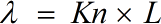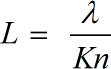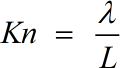Request a Tool

Knudsen Number Calculator

Knudsen Number Calculator is used to calculate the ratio of the mean free path length of the molecules of a fluid to a characteristic length.

Mean Path
0

Formula• λ = Mean Free Path
• Kn = Knudsen Number
• L = Representative Physical Length Scale

Defination / Uses

The dimensionless number is referred to as the Knudsen Number. The Knudsen Number is the ratio of a representative physical length scale to the molecular mean free path length.

Physical Length
0

Formula• L = Representative Physical Length Scale
• Kn = Knudsen Number
• λ = Mean Free Path

Defination / Uses

The dimensionless number is referred to as the Knudsen Number. The Knudsen Number is the ratio of a representative physical length scale to the molecular mean free path length.

Knudsen Number
0

Formula• Kn = Knudsen Number
• L = Representative Physical Length Scale
• λ = Mean Free Path

Defination / Uses

The dimensionless number is referred to as the Knudsen Number. The Knudsen Number is the ratio of a representative physical length scale to the molecular mean free path length.

How to use knudsen number calculator?

There are only two basic steps.

• First select the option from dropdown. What you need to calculate.
• Then add the other values in their corresponding fields.

Thats it! You will get your answer in less than a second. Use upper given formula in case of manual calculation.

In a nutshell, the Knudsen Number is the proportion of a fluid's mean free path length to a characteristic length. It's a term for the movement of low-density gases. The ratio of the mean free path length of a fluid's molecules to a characteristic length is calculated using this advanced online Knudsen number calculator. Our Knudsen number calculator is a straightforward tool for calculating the Knudsen number, which is one of fluid mechanics' dimensionless characteristic values. For quick calculation use our weetools. No sign-up, registration OR captcha is required to use this tool.